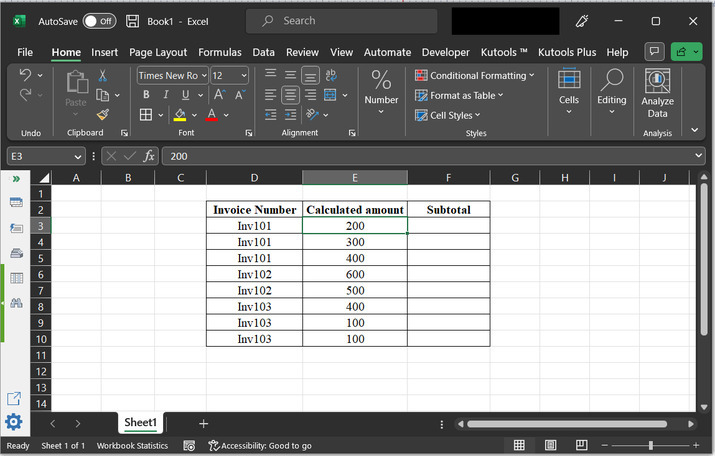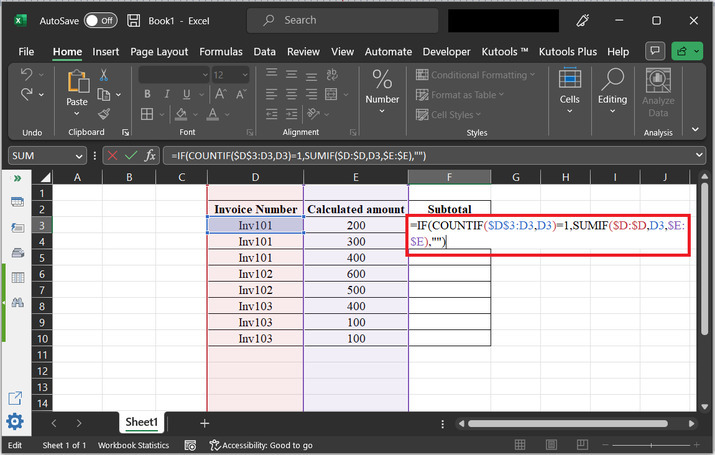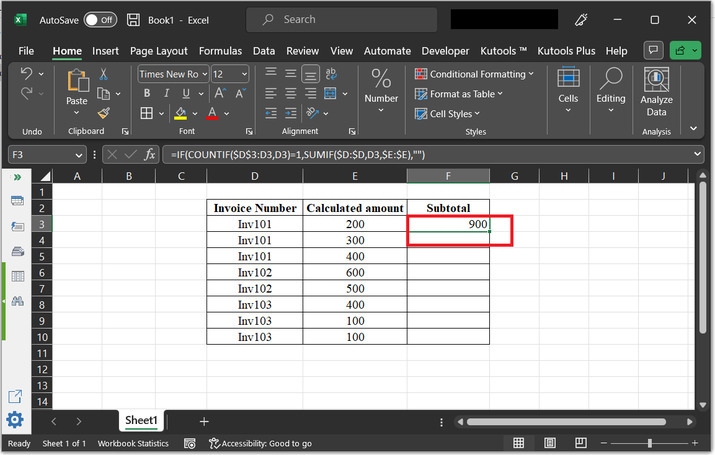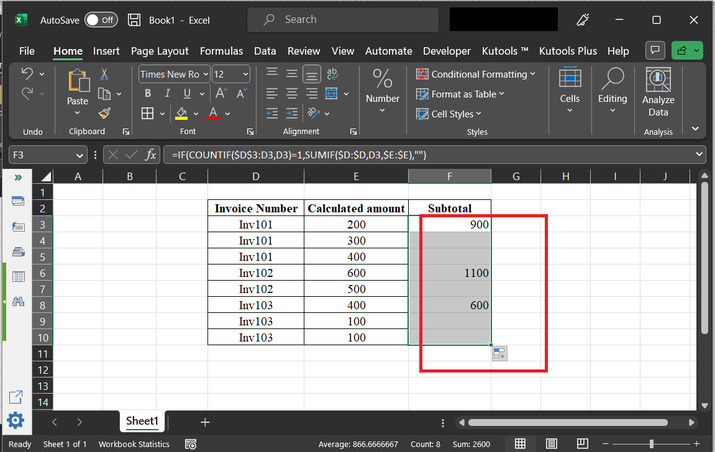# How to get subtotals based on invoice number in Excel?

Calculating the subtotal of an invoice is an important component of an invoice. It allows the user to understand the total amount after putting in the total invoice amount. In this article, the learner is guided through the process of generating a subtotal of the provided invoice number. For example, if invoice 101 has three entries of 100, 200, and 300, then the calculated subtotal amount will become 600. In this article, the user implements the Subtotal value by using the formula, method.

## Example 1: To get subtotals based on invoice numbers in Excel by using the formula:

### Step 1:

To understand the process user, need to follow to calculate subtotals based on the invoice number. Consider below given spreadsheet:### Step 2:

Go to the cell where the user wants to place the calculated subtotal amount. For this case, let’s place the total amount for Inv101 in the F1 cell.

After that in the F1 cell type

“=IF(COUNTIF($D$3:D3,D3)=1,SUMIF($D:$D,D3,$E:$E),"")” and press “Enter key”.

### Snapshot of the implemented formula:### The explanation for the formula:

The main purpose of this formula is to calculate the subtotal for each unique invoice number in a data range that contains the invoice number and the corresponding amounts. The output of the formula will be a list of subtotals for each unique invoice number.

### Consider the breakdown for the working of the formula:

• COUNTIF($D$3:D3,D3) − this part of the formula counts the number of occurrences of the invoice number in the range from D3 to the current row. If the first occurrence of the invoice number is selected then the count will be 1. Otherwise, the count will be greater than 1.

• The IF function checks if the count is equal to 1. If it is, then this is the first occurrence of the invoice number and calculating the subtotal. If it is not equal to 1, then there is no need to calculate the subtotal and the cell will be blank.

• SUMIF($D:$D,D3,$E:$E) − this part calculates the sum of the amounts for the current invoice number. It searches for all occurrences of the current invoice number in column D and sums the corresponding amounts in column E. If the count is equal to 1, then the subtotal is calculated and displayed in the current row. If the count is not equal to 1, then the cell is left blank.

### Step 3

Press the “Enter” key. This will print the output to the F3 cell as highlighted in the below image:### Step 4:

After that click on the right bottom area of the F3 cell, and drag the “+” sign to 10th row. Doing so, will copy the formula to the 10th row. Results are provided below:## Conclusion:

After completing all the steps of this article, the learner will now become capable of generating a subtotal of the provided invoice entries. Simply type the formula, and press the “Enter” key to display the results. Calculating the subtotal of invoices is important. The generated results are accurate and precise. There is no need to check every entry manually, just ensure that the formula copied to all the other cells is generating an accurate result.

Updated on: 17-Apr-2023

239 Views